# LSTM 原理与实践，原来如此简单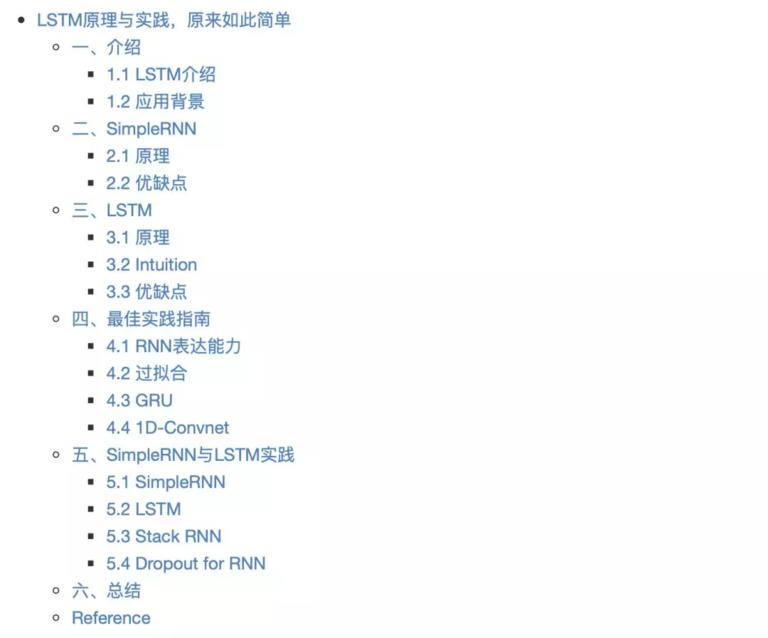## 一、介绍

### 1.1 LSTM 介绍

LSTM 全称 _Long Short-Term Memory_，是 1997 年就被发明出来的算法，作者是谁说了估计也记不住干脆就不说了（主要是我记不住，逃…）

Deep learning is an art more than a science.

1. RNN 原理、应用背景、缺点

2. LSTM 产生原因、原理，以及关于 LSTM 各种“门”的一些 intuition（哲学解释） （别怕，包教包会）

3. 如何利用 Keras 使用 LSTM 来解决实际问题

4. 关于 Recurrent Network 的一些常用技巧，包括：过拟合，stack rnn

### 1.2 应用背景

Recurrent network 的应用主要如下两部分：

1. 文本相关。主要应用于自然语言处理（NLP）、对话系统、情感分析、机器翻译等等领域，Google 翻译用的就是一个 7-8 层的 LSTM 模型。

2. 时序相关。就是时序预测问题（timeseries），诸如预测天气、温度、包括个人认为根本不可行的但是很多人依旧在做的预测股票价格问题

## 二、SimpleRNN

### 2.1 原理

RNN 是 Recurrent Neural Network 的缩写，它就是实现了我们来维护中间信息，记录之前看到信息这样最简单的一个概念的模型。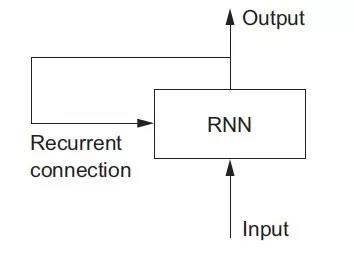``````state_t = 0 #时刻t的状态for input_t in input_sequence: # 在timesteps上loop
output_t = f(input_sequence, state_t) # input_t state_t得到时刻t输出
state_t = output_t # 用当前输出去更新内部状态
``````

`f`是一个函数，它完成从 input 和 state 到 output 的转换，通常包含两个矩阵`W, U`和一个偏置向量`b`，然后再经过激活函数激活。形式如下：

`f = activation(dot(W, input) + dot(U, state) + b)`

``````# SimpleRNN in numpyimport numpy as np

timesteps =  100
input_features =  32
output_features =  64

inputs = np.random.random(shape=(timesteps, input_features))

state_t = np.zeros(shape=(output_features,))  # init state
W = np.random.random(shape=(output_features, input_features))
U = np.random.random(shape=(output_features, output_features))
b = np.random.random(shape=(output_features,))

successive_outputs =  []

for input_t in inputs: output_t = np.tanh(np.dot(W, input_t)  + np.dot(U, state_t)  + b)  #input_t state_t => output_t successive_outputs.append(output_t) state_t = output_t# update state_t using output_t

final_outputs = np.concatenate(successive_outputs, axis=0)  #get the final_output with shape=(timesteps, output_features)
``````### 2.2 优缺点

1. 优点。处理 a sequence 或者 a timeseries of data points 效果比普通的 DNN 要好。中间状态理论上维护了从开头到现在的所有信息；

2. 缺点。不能处理 long sequence/timeseries 问题。原因是梯度消失，网络几乎不可训练。所以也只是理论上可以记忆任意长的序列。

## 三、LSTM

LSTM 就是用来解决 RNN 中梯度消失问题的，从而可以处理 long-term sequences。

### 3.1 原理

LSTM 是 SimpleRNN 的变体，它解决了梯度消失的问题。怎么解决的那？

LSTM 增加了一个可以相隔多个 timesteps 来传递信息的方法。想想有一个传送带在你处理 sequences 时一起运转。每个时间节点的信息都可以放到传送带上，或者从传送带上拿下来，当然你也可以更新传送带上的信息。这样就保存了很久之前的信息，防止了信息的丢失。我们把 SimpleRNN 中的矩阵记为`Wo Uo bo`，LSTM 的结构图如下：`output_t = activation(dot(state_t, Uo) + dot(input_t, Wo) + dot(C_t, Vo) + bo)`

1. RNN 内部的状态 state_t 还是跟之前一样：用上一个时刻的输出来更新。

2. 传送带上的状态更新就是 LSTM 的重点了，也是复杂的地方

``````i_t = activation(dot(state_t, Ui) + dot(input_t, Wi)+ bi)
f_t = activation(dot(state_t, Uf) + dot(input_t, Wf) + bf)
k_t = activation(dot(state_t, Uk) + dot(input_t, Wk) + bk)
``````

`c_t+1 = i_t * k_t + c_t * f_t`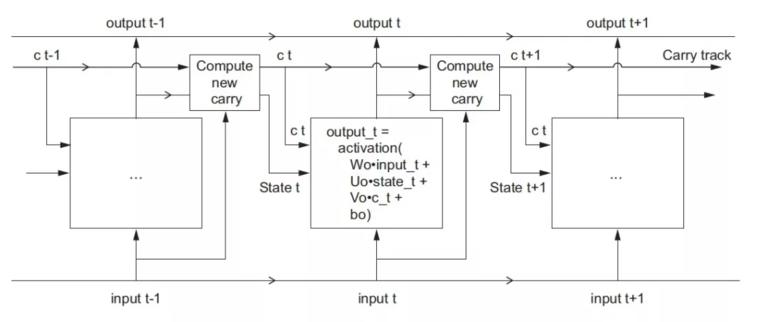### 3.2 Intuition

Deep Learning is an art more than a science.

1. `c_t * f_t` 是为了让模型忘记一些不相关的信息，在 carry dataflow 的时候。即时是很久之前的信息，模型也有不用他的选择权利，所以模型要有忘记不相关信息的能力。 这也就是常说的遗忘门（我觉得翻译成中文真的很没意思，因为中文的“门”意思是在是太多了，你懂得）。

2. `i_t * k_t` 为模型提供关于当前时刻的信息，给 carry track 增加一些新的信息。

### 3.3 优缺点

1. 优点。解决了 SimpleRNN 梯度消失的问题，可以处理 long-term sequence

2. 缺点。计算复杂度高，想想谷歌翻译也只是 7-8 层 LSTM 就知道了；自己跑代码也有明显的感觉，比较慢。

## 四、最佳实践指南

### 4.2 过拟合

RNN LSTM 同样会过拟合。这个问题直到 2015 年，在博士 Yarin Gal 在他的博士论文里给出了解决办法：类似 dropout。但是在整个 timesteps 上使用同一个固定的 drop mask。

### 4.3 GRU

LSTM 的计算比较慢，所有有了 Gated Recurrent Unit（GRU），你可以认为他是经过特殊优化提速的 LSTM，但是他的表达能力也是受到限制的。

### 4.4 1D-Convnet

1. 用 1D-Convnet 来处理简单的文本问题。

2. 把它和 LSTM 融合，利用 1D-Conv 轻量级，计算快的优点来得到低维度特征，然后再用 LSTM 进行学习。这对于处理 long sequence 非常有用，值得尝试。

## 五、SimpleRNN 与 LSTM 实践

1. SimpleRNN

2. LSTM

1. Stack of SimpleRNN

2. Dropout for RNN

### 5.1 SimpleRNN

``````# 5.1 SimpleRNN

from keras.models import Sequential
from keras.layers import Dense, SimpleRNN, Embedding
from keras import datasets
from keras.preprocessing import sequence

max_features = 10000 # 我们只考虑最常用的10k词汇
maxlen = 500 # 每个评论我们只考虑100个单词

(x_train, y_train), (x_test, y_test) = datasets.imdb.load_data(num_words=max_features)
print(len(x_train), len(x_test))

model = Sequential()
model.summary()

model.compile(
optimizer='rmsprop',
loss='binary_crossentropy',
metrics=['acc'])

history = model.fit(
x_train,
y_train,
epochs=10,
batch_size=128,
validation_split=0.2)

plot_acc_and_loss(history)
rets = model.evaluate(x_test, y_test)
print(rets)
``````

validation 的 acc 大约能到 85% 左右。我们只用了 500 个 word 并没有使用全部的 word，而且 SimpleRNN 并不太适合处理 long sequences。期待 LSTM 能有更好的表现.### 5.2 LSTM

``````model = Sequential()
model.summary()
``````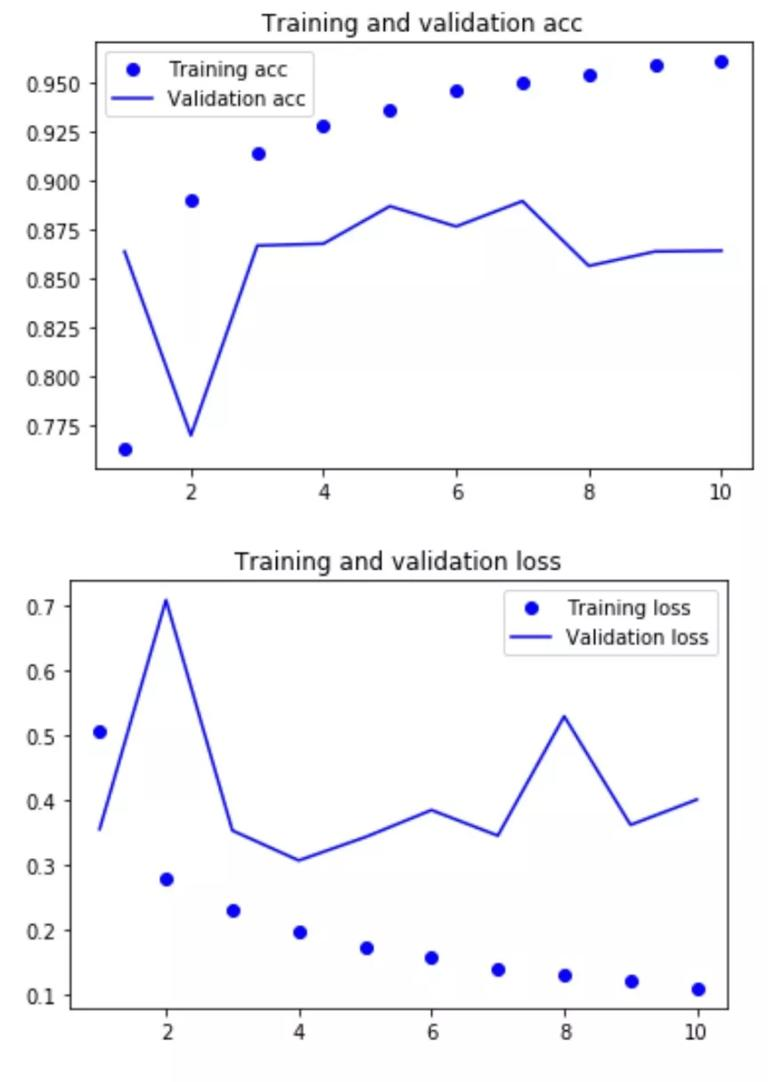### 5.3 Stack RNN

``````model = Sequential()

``````

### 5.4 Dropout for RNN

dropout 同样适用于 lstm layer，留给大家自己去尝试吧。

``````model = Sequential()
``````

## 六、总结

1. LSTM 关键在于增加了 carry track，稍微复杂一点的在于 carry track 上 c_t 信息的更新

2. Recurrent Neural Network 适合 sequence 或 timeseries 问题

3. keras 的 API 非常的人性化，如果是学习或者做实验建议使用 keras，而且 tf 现在也已经内置 keras api 可以通过`from tensorflow import keras`来使用

4. keras 内置 SimpleRNN, LSTM, GRU，同时还可以使用 1D-Conv 来处理 sequence 或 timeseries 问题

5. 可以给 stack RNN 来增加模型的表达能力

6. 可以使用 dropout 来对抗 RNN 的过拟合

## Reference

1. Deep Learning with python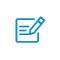扫码入群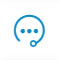咨询反馈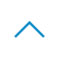返回顶部